# ISEE Middle Level Math : Data Analysis

## Example Questions

### Example Question #91 : Data Analysis

Use the following data set to answer the question: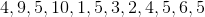Find the range.Explanation:

To find the range of a data set, we will first find the smallest and the largest numbers within the set.  Then, we will find the difference of those numbers.

So, given the setwe can see the smallest number is 1 and the largest number is 10.  Now, we will find the difference.  To find the difference, we will subtract.  So, we get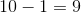Therefore, the range of the data set is 9.

### Example Question #91 : Data Analysis

Use the following data set to answer the question: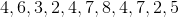Find the range.Explanation:

To find the range of a data set, we will find the smallest and the largest number within the set, then we will find the difference of those two numbers.

So, in the setwe can see the smallest number is 2 and the largest number is 8.  Now, we will find the difference.  To find the difference, we will subtract. So, we get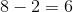Therefore, the range of the data set is 6.

### Example Question #91 : Range

Use the following set to answer the question: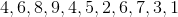Find the range.Explanation:

To find the range of a data set, we will first find the smallest and the largest number within the set.  Then, we will find the difference of those numbers.

So, in the data setwe can see the smallest number is 1 and the largest number is 9.  Now, we will find the difference.  To find the difference, we will subtract the two numbers.  So, we get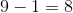Therefore, the range of the data set is.

### Example Question #92 : Range

Use the following data set to answer the question: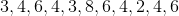Find the range.Explanation:

To find the range of a data set, we will first find the smallest and largest number, then we will find the difference of the two numbers.

So, in the data setwe can see the smallest number is 2 and the largest number is 8.  Now, we will find the difference.  To find the difference, we will subtract the two numbers.  So, we getTherefore, the range of the data set is 6.

### Example Question #93 : Range

Use the following data set to answer the question:Find the range.Explanation:

To find the range of a data set, we will find the smallest and the largest number within the set, then we will find the difference of the two numbers.

So, given the set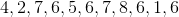we can see the smallest number is 1 and the largest number is 8.  Now, we will find the difference.  To find the difference, we will subtract the two numbers.  So, we get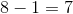Therefore, the range of the data set is 7.

### Example Question #93 : Range

Use the following data set to answer the question: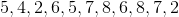Find the range.Explanation:

To find the range of a data set, we will first find the smallest and the largest number within the set.  Then, we will find the difference of those two numbers.

So, given the setWe can see the smallest number is 2 and the largest number is 8.  Now, we will find the difference.  To find the difference, we will subtract.  So,Therefore, the range of the data set is 6.

### Example Question #92 : How To Find Range

Use the following data set to answer the question: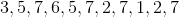Find the range.Explanation:

To find the range of a data set, we will find the smallest and the largest numbers within the set.  Then, we will find the difference of those two numbers.

So, given the setwe can see the smallest number is 1 and the largest number is 7.  Now, we will find the difference.  To find the difference, we will subtract.  We get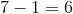Therefore, the range of the data set is 6.

### Example Question #95 : Range

A Math class took an exam.  Here are the scores of 11 students: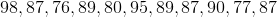Find the range.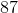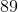Explanation:

To find the range of a data set, we will find the smallest and the largest number.  Then, we will find the difference of those two numbers.

So, given the setWe can see the smallest number is 76 and the largest number is 98.  Now, we will find the difference.  To find the difference, we will subtract the two numbers.  We get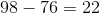Therefore, the range of the data set is 22.

### Example Question #96 : Range

A Science class took an exam.  Here are the scores: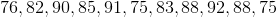Find the range.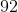Explanation:

To find the range, we will find the smallest and the largest number within the set.  Then, we will find the difference.

So, given the setwe can see the smallest number is 75 and the largest number is 92.  Now, we will find the difference (subtract).

We get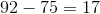Therefore, the range of the data set is 17.

### Example Question #93 : How To Find Range

Use the following data set to answer the question: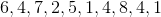Find the range.Explanation:

To find the range, we will find the smallest and the largest number within the set.  Then, we will find the difference of those two numbers.

So, given the setwe can see the smallest number is 1 and the largest number is 8.  Now, we will find the difference.  We getTherefore, the range of the data set is 7.

### All ISEE Middle Level Math Resources